Note: The other languages of the website are Google-translated. Back to English

## 如何基於其他文本突出顯示單元格中的特定文本？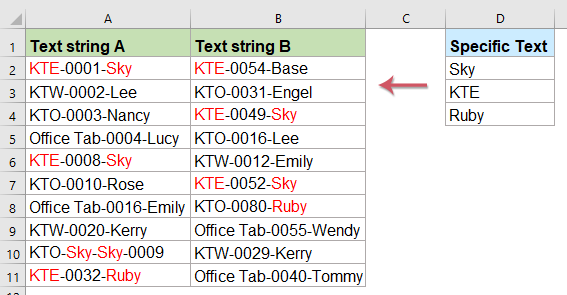#### 使用VBA代碼突出顯示多個單元格中的一個或多個特定文本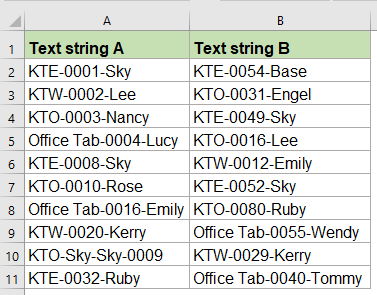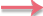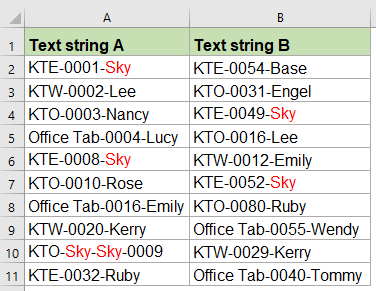1. 選擇要突出顯示特定文本的單元格，然後按住 ALT + F11 鍵打開 Microsoft Visual Basic for Applications 窗口。

2。 點擊 插入 > 模塊，然後將以下代碼粘貼到 模塊 窗口。

VBA代碼：突出顯示單元格中的一部分文本：

``````Sub HighlightStrings()
'Updateby Extendoffice
Application.ScreenUpdating = False
Dim Rng As Range
Dim cFnd As String
Dim xTmp As String
Dim x As Long
Dim m As Long
Dim y As Long
cFnd = InputBox("Enter the text string to highlight")
y = Len(cFnd)
For Each Rng In Selection
With Rng
m = UBound(Split(Rng.Value, cFnd))
If m > 0 Then
xTmp = ""
For x = 0 To m - 1
xTmp = xTmp & Split(Rng.Value, cFnd)(x)
.Characters(Start:=Len(xTmp) + 1, Length:=y).Font.ColorIndex = 3
xTmp = xTmp & cFnd
Next
End If
End With
Next Rng
Application.ScreenUpdating = True
End Sub
``````

3。 然後按 F5 鍵來運行此代碼，然後會彈出一個提示框，提醒您輸入僅要突出顯示的文本，請參見屏幕截圖：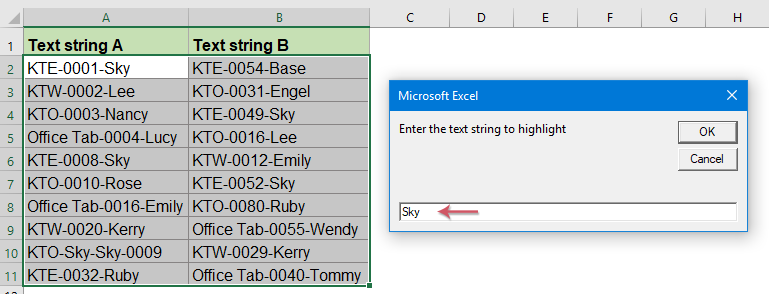4。 然後點擊 OK 按鈕，您指定的所有文本僅在單元格中突出顯示，請參見屏幕截圖：VBA代碼：從文本字符串中突出顯示多個關鍵字：
``````Sub HighlightStrings()
'Updateby Extendoffice
Application.ScreenUpdating = False
Dim Rng As Range
Dim cFnd As String
Dim xTmp As String
Dim x As Long
Dim m As Long
Dim y As Long
Dim xFNum As Integer
Dim xArrFnd As Variant
Dim xStr As String
cFnd = InputBox("Please enter the text, separate them by comma:")
If Len(cFnd) < 1 Then Exit Sub
xArrFnd = Split(cFnd, ",")
For Each Rng In Selection
With Rng
For xFNum = 0 To UBound(xArrFnd)
xStr = xArrFnd(xFNum)
y = Len(xStr)
m = UBound(Split(Rng.Value, xStr))
If m > 0 Then
xTmp = ""
For x = 0 To m - 1
xTmp = xTmp & Split(Rng.Value, xStr)(x)
.Characters(Start:=Len(xTmp) + 1, Length:=y).Font.ColorIndex = 3
xTmp = xTmp & xStr
Next
End If
Next xFNum
End With
Next Rng
Application.ScreenUpdating = True
End Sub
``````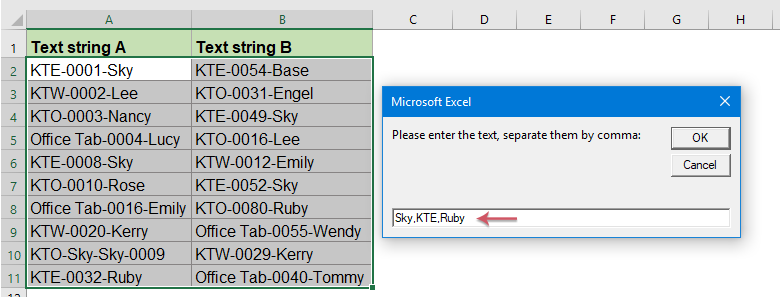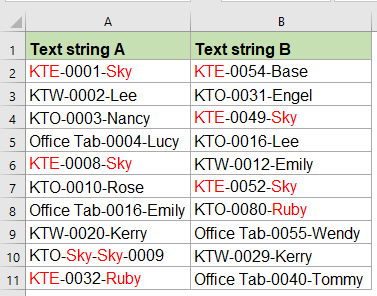#### 以驚人的功能突出顯示多個單元格中的一個或多個特定文本

1。 點擊 庫工具 > 文本 > 標記關鍵字，請參見屏幕截圖：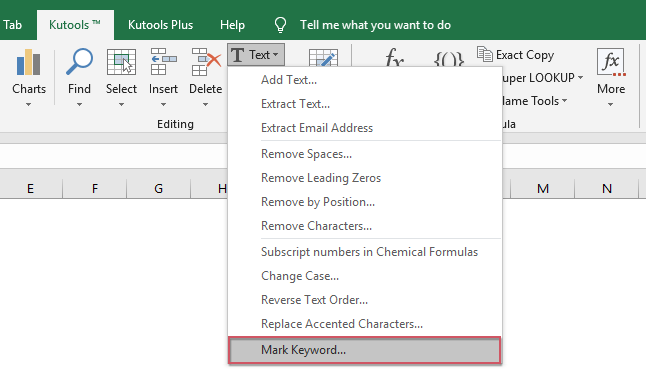2。 在 標記關鍵字 對話框，請執行以下操作：

• 從中選擇要使用的數據范圍 範圍 文本框;
• 選擇包含要突出顯示的關鍵字的單元格，也可以將關鍵字手動輸入（以逗號分隔）到 關鍵詞 文本框
• 最後，您應指定一種字體顏色以通過選中來突出顯示文本 標記關鍵字顏色 選項。 （要為整個包含關鍵字的單元格上色，請選擇 標記單元格內容顏色 選項）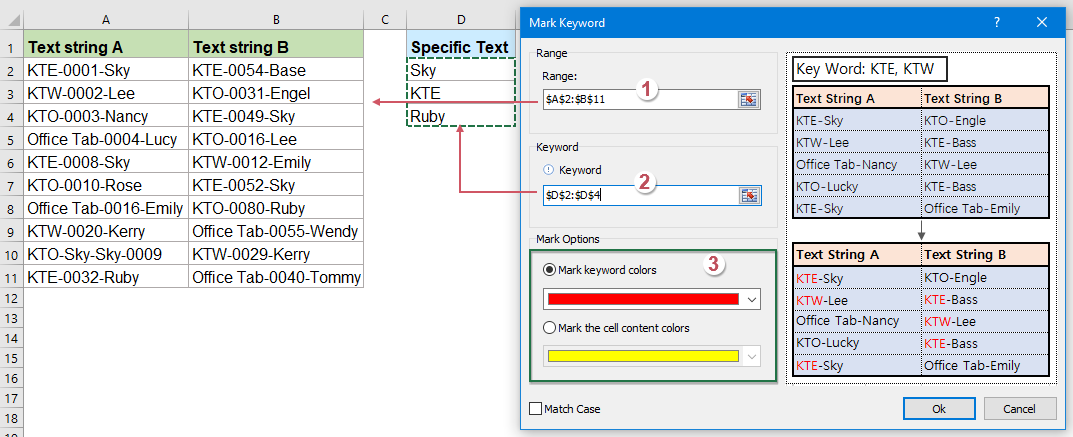3。 然後，點擊 Ok 按鈕，所有指定的文本均已突出顯示，如下圖所示：#### 使用VBA代碼根據其他文本突出顯示單元格中的特定文本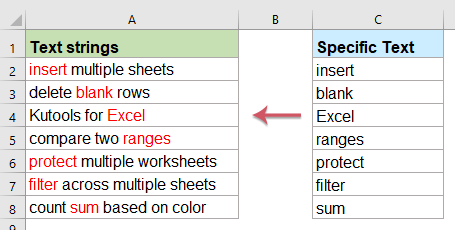1。 按住 ALT + F11 鍵打開 Microsoft Visual Basic for Applications 窗口。

2。 點擊 插入 > 模塊，然後將以下代碼粘貼到 模塊 窗口。

VBA代碼：根據其他文本突出顯示單元格中的部分文本：

``````Sub highlight()
'Updateby Extendoffice
Dim xStr As String
Dim xRg As Range
Dim xTxt As String
Dim xCell As Range
Dim xChar As String
Dim I As Long
Dim J As Long
On Error Resume Next
If ActiveWindow.RangeSelection.Count > 1 Then
Else
End If
LInput:
Set xRg = Application.InputBox("please select the data range:", "Kutools for Excel", xTxt, , , , , 8)
If xRg Is Nothing Then Exit Sub
If xRg.Areas.Count > 1 Then
MsgBox "not support multiple columns"
GoTo LInput
End If
If xRg.Columns.Count <> 2 Then
MsgBox "the selected range can only contain two columns "
GoTo LInput
End If
For I = 0 To xRg.Rows.Count - 1
xStr = xRg.Range("B1").Offset(I, 0).Value
With xRg.Range("A1").Offset(I, 0)
.Font.ColorIndex = 1
For J = 1 To Len(.Text)
If Mid(.Text, J, Len(xStr)) = xStr Then .Characters(J, Len(xStr)).Font.ColorIndex = 3
Next
End With
Next I
End Sub
``````

3。 粘貼代碼後，按 F5 要運行它，請彈出一個提示框，提醒您選擇包含文本字符串和要突出顯示並基於的特定文本的數據范圍，請參見屏幕截圖：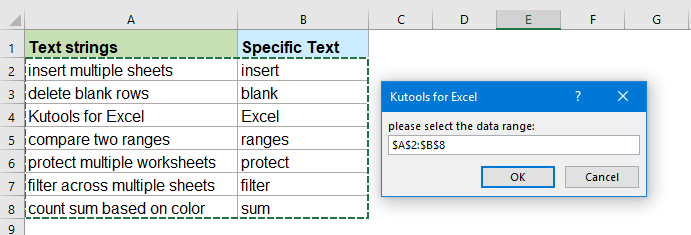4。 然後點擊 OK 按鈕，基於第二列中的特定文本，第一列中的所有相應文本已被染成紅色，如以下屏幕截圖所示：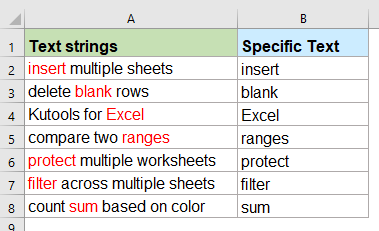#### 更多相關文章：

• 在Excel中連接兩列時的粗體文本
• 在Excel工作表中，將兩個單元格值與公式連接起來後，您可能會發現它不會在組合的公式單元格中加粗文本的一部分。 有時這可能很煩人，當在Excel中連接兩列時如何加粗零件文本？
• 連接單元格列並在Excel中保留文本顏色
• 眾所周知，將單元格列連接或組合為一列時，單元格格式（例如文本字體顏色，數字格式等）將丟失。 本文，我將介紹一些技巧，以將單元格列合併為一個，並在Excel中盡可能輕鬆地保持文本顏色。
• 根據另一列中的值顯示特定的文本
• 假設我有一個數字列表，現在，我想根據此列號在另一列中顯示一些特定的文本。 例如，如果單元格號在1-100之間，則我希望在相鄰的單元格中顯示文本“減少”，如果該數字在101-200之間，則顯示文本“穩定”，並且該數字大於200 ，顯示“ Increase”（增加）文本，如下圖所示。 要在Excel中解決此任務，本文中的以下公式可能會對您有所幫助。
• 在Excel中用文本和數字求和的單元格
• 例如，我有一個包含數字和文本字符串的值列表，現在，我只想對基於相同文本的數字求和，請看以下屏幕截圖。 通常，您不能直接使用文本字符串將列表中的值求和，在這裡，我將向您介紹一些公式來處理此任務。

### 最佳辦公效率工具

#### Kutools for Excel 解決了你的大部分問題，並將你的生產力提高了 80%

• 重用: 快速插入 複雜的公式，圖表 以及您以前使用過的任何東西； 加密單元 帶密碼 創建郵件列表 並發送電子郵件...
• 超級公式欄 （輕鬆編輯多行文本和公式）； 閱讀版式 （輕鬆讀取和編輯大量單元格）； 粘貼到過濾範圍...
• 合併單元格/行/列 不會丟失數據； 拆分單元格內容； 合併重複的行/列...防止細胞重複； 比較範圍...
• 選擇重複或唯一 行; 選擇空白行 （所有單元格都是空的）； 超級查找和模糊查找 在許多工作簿中； 隨機選擇...
• 確切的副本 多個單元格，無需更改公式參考； 自動創建參考 到多張紙； 插入項目符號，複選框等...
• 提取文字，添加文本，按位置刪除， 刪除空間; 創建和打印分頁小計； 在單元格內容和註釋之間轉換...
• 超級濾鏡 （將過濾方案保存並應用於其他工作表）； 高級排序 按月/週/日，頻率及更多； 特殊過濾器 用粗體，斜體...
• 結合工作簿和工作表; 根據關鍵列合併表； 將數據分割成多個工作表; 批量轉換xls，xlsx和PDF...
• 超過 300 項強大的功能. 支持 Office / Excel 2007-2021 和 365。支持所有語言。 在您的企業或組織中輕鬆部署。 完整功能 30 天免費試用。 60 天退款保證。#### Office選項卡為Office帶來了選項卡式界面，使您的工作更加輕鬆

• 在Word，Excel，PowerPoint中啟用選項卡式編輯和閱讀，發布者，Access，Visio和Project。
• 在同一窗口的新選項卡中而不是在新窗口中打開並創建多個文檔。
• 將您的工作效率提高 50%，每天為您減少數百次鼠標點擊！.Characters(開始:=Len(xTmp) + 1, 長度:=y).Font.ColorIndex = 3

“在所有文本字符串中查找並標記關鍵字 1、關鍵字 2 和關鍵字 3，即使一個字符串包含多個這些關鍵字（所有這些關鍵字都應在特定文本字符串中突出顯示）”

Application.ScreenUpdating = False

cFnd = InputBox("請輸入文字，用逗號隔開：")

xArrFnd = 拆分（cFnd，“，”）

xStr = xArrFnd(xFNum)
y = Len(xStr)
m = UBound（拆分（Rng.Value，xStr））

xTmp = ""

xTmp = xTmp & Split(Rng.Value, xStr)(x)
.Characters(開始:=Len(xTmp) + 1, 長度:=y).Font.ColorIndex = 3
xTmp = xTmp & xStr

Application.ScreenUpdating = True
END SUB

``````Sub HighlightStrings()
'Updateby Extendoffice
Application.ScreenUpdating = False
Dim Rng As Range
Dim cFnd As String
Dim xTmp As String
Dim x As Long
Dim m As Long
Dim y As Long
Dim xFNum As Integer
Dim xArrFnd As Variant
Dim xStr As String
cFnd = InputBox("Please enter the text, separate them by comma:")
If Len(cFnd) < 1 Then Exit Sub
xArrFnd = Split(UCase(cFnd), ",")
For Each Rng In Selection
With Rng
For xFNum = 0 To UBound(xArrFnd)
xStr = xArrFnd(xFNum)
y = Len(xStr)
m = UBound(Split(UCase(Rng.Value), UCase(xStr)))
If m > 0 Then
xTmp = ""
For x = 0 To m - 1
xTmp = xTmp & Split(UCase(Rng.Value), UCase(xStr))(x)
.Characters(Start:=Len(xTmp) + 1, Length:=y).Font.ColorIndex = 3
xTmp = xTmp & xStr
Next
End If
Next xFNum
End With
Next Rng
Application.ScreenUpdating = True
End Sub``````

Application.ScreenUpdating = False

cFnd = InputBox("請輸入文字，用分號隔開：")

xArrFnd = 拆分（cFnd，“;”）

xStr = xArrFnd(xFNum)
y = Len(xStr)
m = UBound（拆分（Rng.Value，xStr））

xTmp = ""

xTmp = xTmp & Split(Rng.Value, xStr)(x)
.Characters(開始:=Len(xTmp) + 1, 長度:=y).Font.ColorIndex = 3
xTmp = xTmp & xStr

Application.ScreenUpdating = True
END SUB

Application.ScreenUpdating = False

cFnd = InputBox("請輸入文字，用冒號隔開：")

xArrFnd = 拆分（cFnd，“：”）

xStr = xArrFnd(xFNum)
y = Len(xStr)
m = UBound（拆分（Rng.Value，xStr））

xTmp = ""

xTmp = xTmp & Split(Rng.Value, xStr)(x)
.Characters(開始:=Len(xTmp) + 1, 長度:=y).Font.ColorIndex = 3
xTmp = xTmp & xStr

Application.ScreenUpdating = True
END SUB

Set xRg = Application.InputBox("請選擇數據范圍：", "Kutools for Excel", xTxt, , , , , 8)

MsgBox "不支持多列"

MsgBox "所選範圍只能包含兩列"

xStr = xRg.Range("B1").Offset(i, 0).Value

.Font.ColorIndex = 1

.Characters(J, Len(xStr)).刪除

END SUB

HI

0   字符

## 關注我們

Microsoft和Office徽標是Microsoft Corporation在美國和/或其他國家的商標或註冊商標。